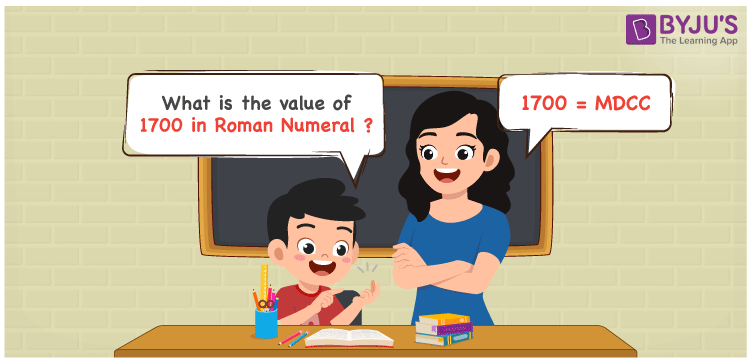# 1700 in Roman Numerals

MDCC is 1700 in Roman numerals. We shall write 1700 in the extended form, i.e. 1700 = 1000 + 500 + 100 + 100 in Roman Numerals. 1700 = M + D + C + C = MDCC is obtained by substituting the converted numbers with their appropriate roman numerals. In this article, we’ll show you how to convert 1700 to Roman numerals correctly.

 Number Roman Numeral 1700 MDCC## How to Write 1700 in Roman Numerals?

In order to write 1700 in Roman Numerals, it has to be written in expanded form, i.e.

1700 = 1000 + 500 + 100 + 100

1700 = M + D + C + C

1700 = MDCC

## Video Lesson on Roman Numerals## Frequently Asked Questions on 1700 in Roman Numerals

Q1

### Write 1700 in Roman Numerals.

In Roman Numerals, 1700 is written as MDCC.
Q2

### How to convert 1700 into Roman Numerals?

The conversion of 1700 into Roman Numerals includes breaking the number according to its place value.
Thousands = 1000 = M
Hundreds = 700 = DCC
Number = 1000 + 700 = M + DCC = MDCC
Q3

### Why is 1700 in Roman Numerals Written as MDCC?

We know that in roman numerals, we write 500 as D, 100 as C, and 1000 as M.
Therefore, 1700 in roman numerals is written as 1700 = 1000 + 700 = M + DCC = MDCC.